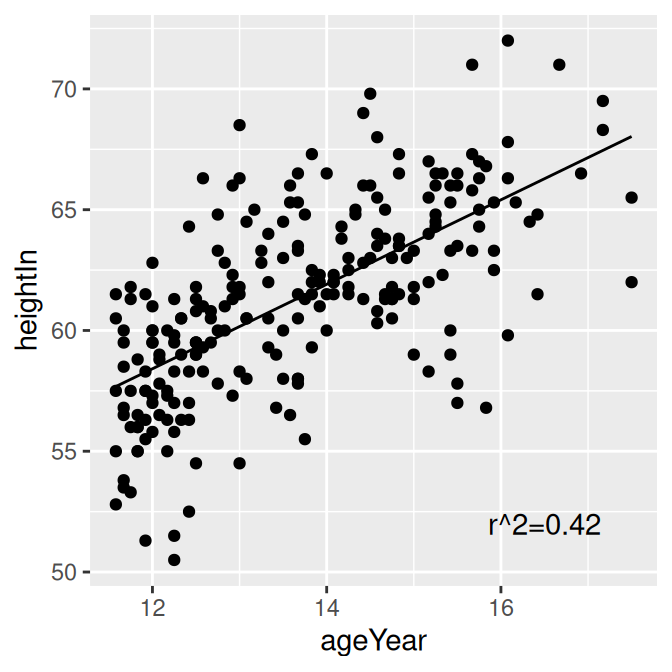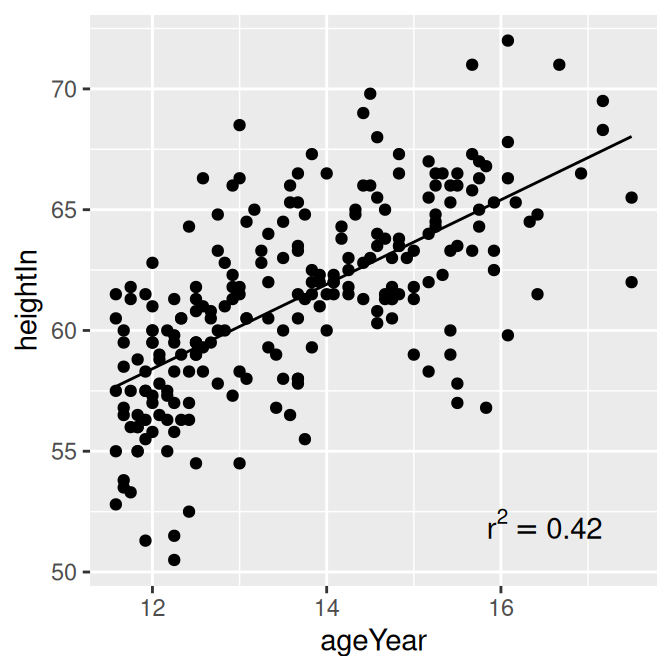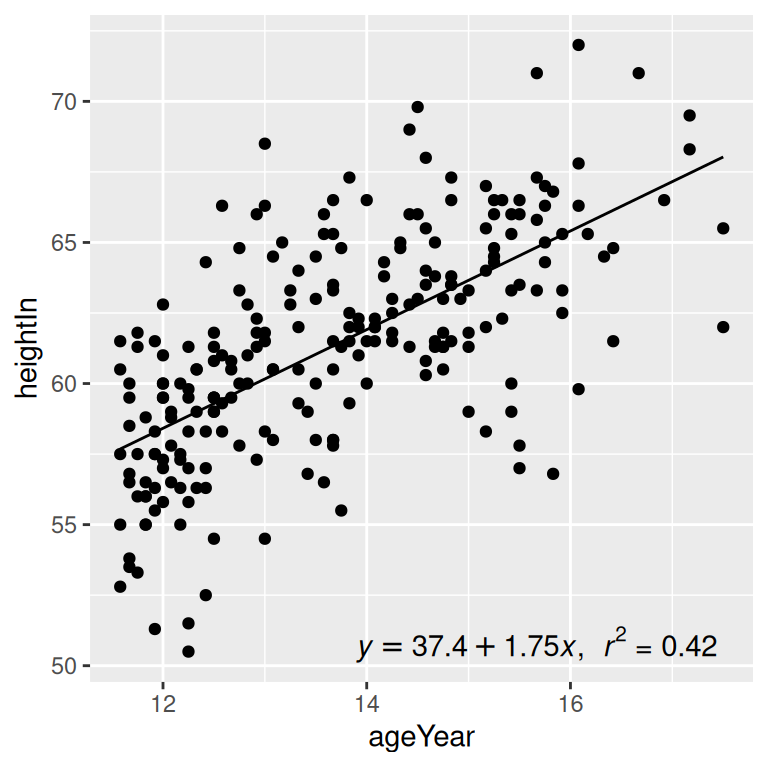## 5.9 Adding Annotations with Model Coefficients

### 5.9.1 Problem

You want to add numerical information about a model to a plot.

### 5.9.2 Solution

To add simple text to a plot, simply add an annotation. In this example, we’ll create a linear model and use the `predictvals()` function defined in Recipe 5.7 to create a prediction line from the model. Then we’ll add an annotation:

``````library(gcookbook) # Load gcookbook for the heightweight data set

model <- lm(heightIn ~ ageYear, heightweight)
summary(model)
#>
#> Call:
#> lm(formula = heightIn ~ ageYear, data = heightweight)
#>
#> Residuals:
#>     Min      1Q  Median      3Q     Max
#> -8.3517 -1.9006  0.1378  1.9071  8.3371
#>
#> Coefficients:
#>             Estimate Std. Error t value Pr(>|t|)
#> (Intercept)  37.4356     1.8281   20.48   <2e-16 ***
#> ageYear       1.7483     0.1329   13.15   <2e-16 ***
#> ---
#> Signif. codes:  0 '***' 0.001 '**' 0.01 '*' 0.05 '.' 0.1 ' ' 1
#>
#> Residual standard error: 2.989 on 234 degrees of freedom
#> Multiple R-squared:  0.4249, Adjusted R-squared:  0.4225
#> F-statistic: 172.9 on 1 and 234 DF,  p-value: < 2.2e-16``````

This shows that the r^2 value is 0.4249. We’ll create a graph and manually add the text using `annotate()` (Figure 5.26):

``````# First generate prediction data
pred <- predictvals(model, "ageYear", "heightIn")

# Save a base plot
hw_sp <- ggplot(heightweight, aes(x = ageYear, y = heightIn)) +
geom_point() +
geom_line(data = pred)

hw_sp +
annotate("text", x = 16.5, y = 52, label = "r^2=0.42")``````

Instead of using a plain text string, it’s also possible to enter formulas using R’s math expression syntax, by using `parse = TRUE`:

``````hw_sp +
annotate("text", x = 16.5, y = 52, label = "r^2 == 0.42", parse = TRUE)``````Figure 5.26: Plain text (left); Math expression (right)

### 5.9.3 Discussion

Text geoms in ggplot do not take expression objects directly; instead, they take character strings that can be turned into expressions with R’s `parse()` function.

If you use a mathematical expression, the syntax must be correct for the expression to be a valid R expression object. You can test the validity by wrapping the object in the `expression()` function and seeing if it throws an error (make sure not to use quotes around the expression). In the example here, `==` is a valid construct in an expression to express equality, but `=` is not:

``````expression(r^2 == 0.42) # Valid
expression(r^2 = 0.42)  # Not valid
#> Error: unexpected '=' in "expression(r\^2 ="``````

It’s possible to automatically extract values from the model object and build an expression using those values. In this example, we’ll create a string which when parsed, yields a valid expression:

``````# Use sprintf() to construct our string.
# The %.3g and %.2g are replaced with numbers with 3 significant digits and 2
# significant digits, respectively. The numbers are supplied after the string.

eqn <- sprintf(
"italic(y) == %.3g + %.3g * italic(x) * ',' ~~ italic(r)^2 ~ '=' ~ %.2g",
coef(model),
coef(model),
summary(model)\$r.squared
)

eqn
#>  "italic(y) == 37.4 + 1.75 * italic(x) * ',' ~~ italic(r)^2 ~ '=' ~ 0.42"

# Test validity by using parse()
parse(text = eqn)
#> expression(italic(y) == 37.4 + 1.75 * italic(x) * "," ~ ~italic(r)^2 ~
#>     "=" ~ 0.42)``````

Now that we have the expression string, we can add it to the plot. In this example we’ll put the text in the bottom-right corner, by setting `x = Inf` and `y = -Inf` and using horizontal and vertical adjustments so that the text all fits inside the plotting area (Figure 5.27):

``````hw_sp +
annotate(
"text",
x = Inf, y = -Inf,
label = eqn, parse = TRUE,
hjust = 1.1, vjust = -.5
)``````Figure 5.27: Scatter plot with automatically generated expression